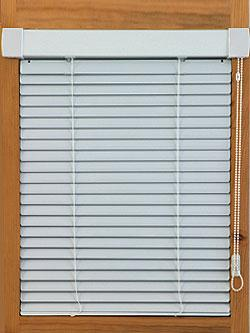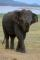# Louvers

The company charges for office equipment louvers total 262 Eur. From the delivery bill is obvious that the louvers are 76 Eur expensive than their installation.

What percentage of the total charged is the installation of louvers?

Correct result:

p =  35.5 %

#### Solution:

$z+m =262 \ \\ z = 76 + m \ \\ p = \dfrac{m}{x}\cdot 100 \ \\ p = \dfrac{ 262-76}{ 2\cdot 262 } \cdot 100 = 35.5 \%$

z+m =262
z = 76 + m

m+z = 262
m-z = -76

m = 93
z = 169

Our linear equations calculator calculates it.We would be pleased if you find an error in the word problem, spelling mistakes, or inaccuracies and send it to us. Thank you!Tips to related online calculators
Check out our ratio calculator.
Do you have a linear equation or system of equations and looking for its solution? Or do you have quadratic equation?

## Next similar math problems:

• Percentage - fractionsAbout what percentage we must increase number 1/6 to get number 1/3?
• GlovesI have a box with two hundred pieces of gloves in total, split into ten parcels of twenty pieces, and I sell three parcels. What percent of the total amount I sold?
• ClassIn a class are 32 pupils. Of these are 8 boys. What percentage of girls are in the class?
• NumberWhat number is 20 % smaller than the number 198?
• Conference148 is the total number of employees. The conference was attended by 22 employees. How much is it in percent?
• Apples 2James has 13 apples. He has 30 percent more apples than Sam. How many apples has Sam?
• 100 %How much is 100%, if 17 % is 1169?
• ClassIn 7.C clss are 10 girls and 20 boys. Yesterday was missing 20% of girls and 50% boys. What percentage of students missing?
• EquationSolve the equation: 1/2-2/8 = 1/10; Write the result as a decimal number.
• Unknown numberIdentify unknown number which 1/5 is 40 greater than one tenth of that number.
• 1.5 divided1.5 divided by 1 = w divided by 4
• Sales offGoods is worth € 70 and the price of goods fell two weeks in a row by 10%. How many % decreased overall?
• Percents - easyHow many percent is 432 out of 434?
• Simple equationSolve for x: 3(x + 2) = x - 18
• Seeds 2How many seeds germinated from 1000 pcs, when 23% no emergence?
• IronIron ore contains 57% iron. How much ore is needed to produce 20 tons of iron?
• Base, percents, valueBase is 344084 which is 100 %. How many percent is 384177?